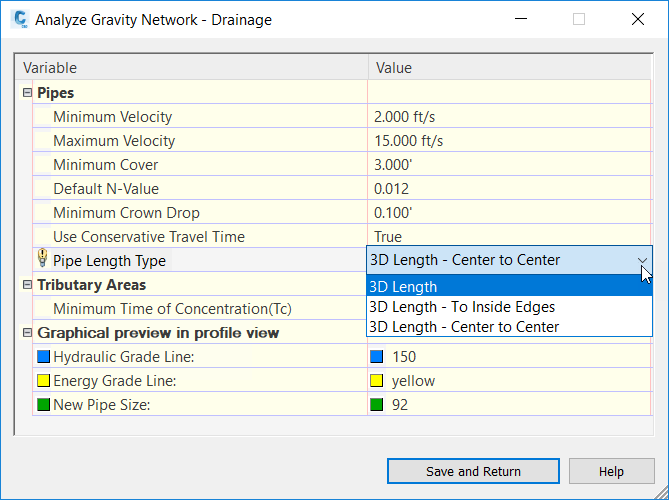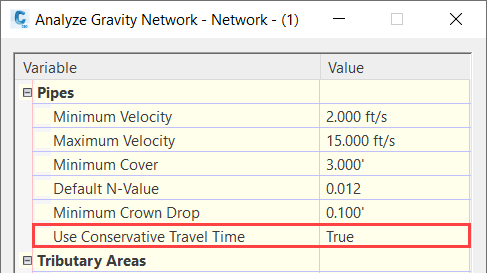# Gravity Network Analysis Enhancements

## New in 2020.6

Gravity network analysis has a new setting called Pipe Length Type which you can use to specify the pipe length type to use for the analysis calculations.## New in 2020.5

Gravity network analysis has a new setting named Use Conservative Travel Time that you can use to specify whether the minimum velocity or the calculated design velocity will be used to calculate the pipe section time if the design velocity is smaller than the minimum velocity.If the calculated design velocity is larger than the minimum velocity, the design velocity will be used to calculate section time whether Use Conservative Travel Time is set to True or False.

If the design velocity is smaller than the minimum velocity:

• When Use Conservative Travel Time is set to True, the minimum velocity will be used to calculate the pipe section time.
• When Use Conservative Travel Time is set to False, the design velocity will be used to calculate the pipe section time.